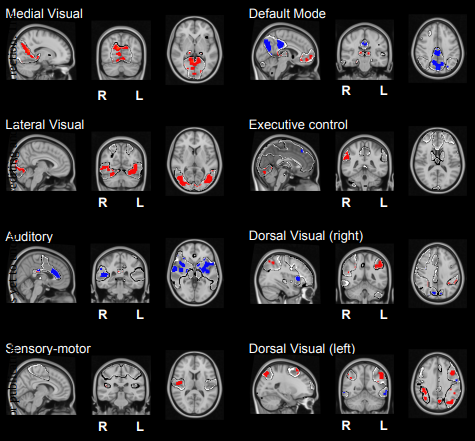Brain Connectivity & Machine Learning# Information flow between resting state networks

I. Diez*, A. Erramuzpe*, I. Escudero, B. Mateos, D. Marinazzo, E. Sanz-Arigita, S. Stramaglia and J.M. Cortes (* Equal Contribution). Information flow between resting state networks. Brain Connectivity 5:554-564, 2015 [pdf] [arXiv:1505.03560]
The resting brain dynamics self-organizes into a finite number of correlated patterns known as resting state networks (RSNs). It is well known that techniques like independent component analysis can separate the brain activity at rest to provide such RSNs, but the specific pattern of interaction between RSNs is not yet fully understood. To this aim, we propose here a novel method to compute the information flow (IF) between different RSNs from resting state magnetic resonance imaging. After hemodynamic response function blind deconvolution of all voxel signals, and under the hypothesis that RSNs define regions of interest, our method first uses principal component analysis to reduce dimensionality in each RSN to next compute IF (estimated here in terms of Transfer Entropy) between the different RSNs by systematically increasing k (the number of principal components used in the calculation). When k equal to 1, this method is equivalent to computing IF using the average of all voxel activities in each RSN. For k bigger than 1 our method calculates the k-multivariate IF between the different RSNs. We find that the average IF among RSNs is dimension-dependent, increasing from k equal to 1 (i.e., the average voxels activity) up to a maximum occurring at k equal to 5 to finally decay to zero for k bigger than 10. This suggests that a small number of components (close to 5) is sufficient to describe the IF pattern between RSNs. Our method — addressing differences in IF between RSNs for any generic data — can be used for group comparison in health or disease. To illustrate this, we have calculated the inter-RSNs IF in a dataset of Alzheimer’s Disease (AD) to find that the most significant differences between AD and controls occurred for k equal to 2, in addition to AD showing increased IF w.r.t. controls. The spatial localization of the k equal to 2 component, within-RSNs, allows the characterization of IF differences between Alzheimer‘s and controls.

This website uses its own cookies for its proper functioning and better user experience. By navigating this website and/or clicking the Accept button, you agree to the use of these technologies and the processing of your data for these purposes. More information    Privacy policy